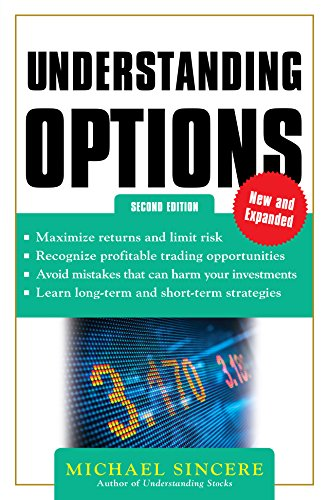Online Calculators > Financial Calculators > Options Calculator

# Options Calculator

Options Calculator to calculate profit or loss for any call and put option. Options profit calculator calculates the total returns for your options trading based on options price, number of contracts, current stock price, strike price and expected stock price.

Select "call" to use call option calculator or choose "put" to use put option calculator.

## Options Profit Calculator

Option Price Paid \$
# of Contract
Current Stock Price \$
Strike Price/Exercise Price \$
Expected Stock Price \$
Call or Put?
 Initial Investment Intrinsic Value Final Option Price Final Investment Value Total Profit

Shares
Purchase Price
Sell Price
Initial Investment
Final Stock Value
Total Profit
Return On Investment

# Options Profit Calculator

Options profit calculator will compare your initial cost and final return on investment if you were buying the stock rather than options.

To learn how to trade options for profit, you may want to read the following three books on how to trade and invest in options.
1. The Options Playbook2. Understanding Options 2EElectrical Calculators
Real Estate Calculators
Accounting Calculators
Construction Calculators
Sports Calculators

Financial Calculators
Compound Interest Calculator
Mortgage Calculator
How Much House Can I Afford
Loan Calculator
Stock Calculator
Options Calculator
Investment Calculator
Retirement Calculator
401k Calculator
eBay Fee Calculator
PayPal Fee Calculator
Etsy Fee Calculator
Markup Calculator
TVM Calculator
LTV Calculator
Annuity Calculator
How Much do I Make a Year

Math Calculators
Mixed Number to Decimal
Ratio Simplifier
Percentage Calculator

Health Calculators
BMI Calculator
Weight Loss Calculator

Conversion
CM to Feet and Inches
MM to Inches

Others
How Old am I
Random Name Picker
Random Number Generator
Multiplication Chart